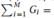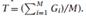### Create an Account

Home / Questions / Status-quo bias Fernandez and Rodrik 1991 There are two possible policies A and B Each ind...

# Status-quo bias Fernandez and Rodrik 1991 There are two possible policies A and B Each individual is either one unit of utility better off under Polcy A or one unit worse off Fraction f

Status-quo bias. (Fernandez and Rodrik, 1991.) There are two possible policies, A and B. Each individual is either one unit of utility better off under Policy A or one unit worse off. Fraction f of the population knows what its welfare would be under each policy. Of these individuals, fraction α are better off under Policy A and fraction 1 − α are worse off. The remaining individuals in the population know only that fraction β of them are better off under Policy A and fraction 1 − β are worse off.

A decision of whether to adopt the policy not currently in effect is made by majority vote. If the proposal passes, all individuals learn which policy makes them better off; a decision of whether to revert to the original policy is then made by majority vote. Each individual votes for the policy that gives him or her the higher expected utility. But if the proposal to revert to the original policy would be adopted in the event that the proposal to adopt the alternative policy passed, no one votes for the alternative policy. (This assumption can be justified by introducing a small cost of changing policies.)

(a) Find an expression for the fraction of the population that prefers Policy A (as a function of f , α, and β) for the case where fraction 1 − f of the population knows only that fraction β of them are better off under Policy A.

(b) Find the analogous expression for the case where all individuals know their welfare under both policies.

(c) Given your answers to parts (a) and (b), can there be cases when whichever policy is initially in effect is retained?

The common-pool problem in government spending. (Weingast, Shepsle, and Johnsen, 1981.) Suppose the economy consists of M > 1 congressional districts. The utility of the representative person living in district i is E +V(Gi)−C (T ). E is the endowment, Gi is the level of a local public good in district i, and T is taxes (which are assumed to be the same in all districts). Assume V’(•) > 0, V”(•) < 0, C’(•) > 0, and C “(•) > 0. The government budget constraint isMT. The representative from each district dictates the values of G in his or her district. Each representative maximizes the utility of the representative person living in his or her district.

(a) Find the first-order condition for the value of Gj chosen by the representative from district j, given the values of Gi chosen by the other representatives and the government budget constraint (which implies(Note: Throughout, assume interior solutions.)

(b) Find the condition for the Nash equilibrium value of G. That is, find the condition for the value of G such that if all other representatives choose that value for their Gi , a given representative wants to choose that value.

(c) Is the Nash equilibrium Pareto-efficient? Explain. What is the intuition for this result?

May 01 2020 View more View LessSubscribe To Get Solution